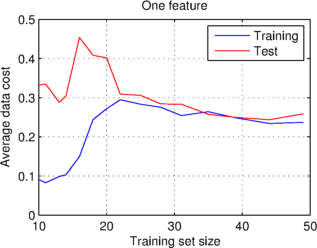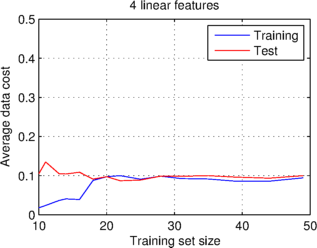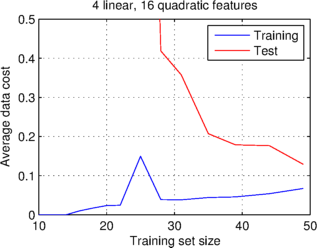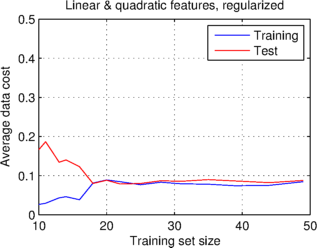# Analysing Learning Curves

I recently looked over Machine Learning high-flier Andrew Ng's Machine Learning course on Coursera. It's perfect for people who want to use machine learning as a tool, and is also a great primer for people who want to get into machine learning research, though it is far more vocational than theoretical.

Of course, being practical, it has all sorts of useful tips to help you get your methods working well. One such tip is some basic plotting and analysis of the learning curves of your current implementation, which can point you towards what to try next if things aren't quite working yet. This analysis can save you a lot of time! I recommend the course, but if you just want to know how to do this analysis then look no further than this blog post.

## Data, model and learning

For any machine learning problem, you should have some training data, and a model you want to learn, or fit to the data. For example, in this post I'll be using a dataset of fuel octane rating versus raw materials, and the model I'll be fitting to this data is a linear function which takes as input the raw material composition, and outputs the real-valued octane rating - this is known as linear regression.

The model defines a cost function to minimize. This generally consists of a term which indicates how well the model agrees with the data, called the data cost, and possibly one or more other terms, which can regularize the space of solutions. Learning is then the process of minimizing the cost function over the training set, with respect to the model parameters. All very standard thus far. In addition, you need to split your data up into two sets, one for learning the model parameters (the "training" set), and one for evaluating them (the "test" set). That's fairly common too.

## Learning curves

Learning curves are created as follows:

1. Learn the model parameters for several different sizes of training data sets, upto the largest size possible (whilst leaving enough data aside for the test set).
2. For each set of learned parameters, compute the average data cost (note that this does not include any regularization costs) per input datum used to learn the parameters, and per test set datum.
3. Plot the average data costs for both the training set and the test set, plotted against the training set size.

I have done this below, using the example dataset I mentioned earlier, for two cases, the first using just one material composition value (or data feature), and the second using all four values (or features) given in the dataset.What the graphs show is that at a certain training set size, the average data costs converge on a stable value, which is the same for the training and test sets. They also show that the learning curves for the case of just one feature have a significantly higher data cost than the other case.

## Bias and variance

Bias is the name given to a constant error, in the case of learning curves an error which affects both the training and test sets. The first graph above exhibits high bias, because both curves settle on a large average data cost, relative to what we might expect or desire. High bias is caused by the model underfitting the data; the particular bias above caused by the model not having enough features to fit to. By contrast, the second graph, where the model has more features to use, has a lower bias, within the realms of what one might wish for.

Variance is the name given to a variable error, in the case of learning curves an error which affects the test set but not the training set. Below I have plotted two further pairs of learning curves. In the case of the first graph I have used even more features, by including all quadratic combinations of features, giving twenty features in total. What you can see is that the data costs do not quite stabilize as the training set size increases, though they look like they could converge with more data. In addition, the data cost of the test set is significantly higher than that of the training set, so this graph exhibits high variance.High variance is caused by the model overfitting the training data, and therefore not transferring well to the test data. Overfitting can be caused by having many unnecessary features (as is likely in the case above), or not enough training data. One way of reducing overfitting is to use a regularization term in the cost function, which prefers simpler models. I have used a quadratic cost on the model parameters to regularize the model, whose learning curves are shown in the second graph above. This graph exhibits low variance, indicating that the model is now not overfit.

## What steps to take

When you look at your learning curves, you should see one of three scenarios:

1. The test set data cost is higher than the training set data cost (high variance), indicating that the model is overfitting the data. Possible steps to try in this case are:
1. Using more training data.
2. Using fewer data features.
3. Using a regularization term which prefers simpler models.
2. The data costs are similar, but higher than desirable (high bias), indicating that the model is underfitting the data. Possible steps to try in this case are:
1. Using more data features, either by taking more measurements or by creating features by multiplying together those already given.
2. Using a more complex model, e.g. by changing from linear regression to a neural net.
3. The data costs are similar, and sufficiently low, indicating low variance and low bias. In this case you're all done!

I hope that is useful. Code for generating these figures can be found in biasvarianceanalysis_demo.m from our ml_examples GitHub repository.